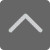爱智康资讯站

课程咨询: 4000-121-121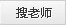二年级上册数学期末试卷-小学数学二年级上册第二单元测试题

2019-01-09 23:12:34 　来源：网络整理

二年级上册数学期末试卷-小学数学二年级上册第二单元测试题你已经二年级了，你对数学科目的兴趣变了吗？下面就是小编为大家带来的二年级上册数学期末试卷-小学数学二年级上册第二单元测试题，希望可以帮助到大家。

一、口算(19分)

1、50+20= 70+5= 12-7= 78-70= 62-7=

65+8= 6+55= 90-40= 75-25= 54-14=

67-56= 56-9= 42+9= 43+3= 48+4=

25+3= 9+38= 60-35= 89-80= 43+27=

40-17= 30+22= 78-50= 28+32= 45-23=

67-24= 65-6= 77-34= 96-61= 49+5=

25+( )=30 ( )+62=70 77-( )=5 ( )-8=20

45-25-3= 45+40-35= 43-6-9= 100-70+5=

二、填一填(27分)

1、我会在计数器上画线表示出结果，并填空。(2分)

2、在○里填上“>、<”或“=”(3分)

42-8○45-8 6+27○33 25+33○52

7+19○19+7 88-19○68 46-12+28○46+17

3、找规律填数。(3分)

(1)28，35，42，( )，( )，63，( )

(2)80，72，( )，56，( )，( )，32

4、(3分)

“蒙牛”牌酸奶 “友芝友”牌酸奶 “光明”牌酸奶

原有 86盒 ( )盒 70盒

卖出 29盒 28盒 ( )盒

剩下 ( )盒 46盒 35盒

5、( )里最大能填几。(3分)

32+( )<40 ( )+25<30 ( )-6<50

80-( )>50 ( )+30<47 92-( )>38

6、放卡片(5分)

31+25 90-52 18+39 25+8 34+28

48-16 27+6 22+43 96+57 39+14

得数小于50的卡片 得数大于50的卡片

三、笔算下列各题(24分)

54-19= 38+24= 75-46= 33+55=

34+12+17= 80-13-37= 67-28+34= 46+17-25=

44+28+26= 85-27-35= 48+29-39= 80-21+39=

四、解决问题。(30分)

1、妈妈买来36个苹果，第一天吃了16个，还剩多少个?(2分)

2、有两盒粉笔，第一盒有45枝，第二盒有37枝，两盒一共有多少枝?(2分)

3、工程对修一条长40米的水泥路，第一天修了15米，第二天比第一天多修了9米，第二天修了多少米?(3分)

4、学校合唱队，男生26人，女生比男生多9人。(4分)

(1)女生有多少人?

(2)合唱队一共有多少人?

5、鸡32只， 鸭16只 ， 鹅24只

(1)鸡比鸭多多少只?(2分) (2)鸡、鸭、鹅共有多少只?(2分)

6、 一辆大客车，车上一共有70个座位，已经坐了45人，又上来了3人。还有几个座位空着?(3分)

小编推荐：

以上就是小编特意为大家整理的二年级上册数学期末试卷-小学数学二年级上册第二单元测试题希望对有需要的同学提供帮助，大家如果在学习中有什么疑问，欢迎拨打爱智康免费电话：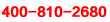!那里有专业的老师为大家解答。

2019年精品学习包：

•小学语|数|英

历年试卷、北京30所校练习题、名著|重点校巡礼|等100讲视频、纸质书籍领取

•初中九大学科

历年真题、期末|期中|志愿填报|重点校巡礼|等100讲视频、纸质书籍领取

•高中九大学科

历年真题、期末|期中|志愿填报|重点校巡礼|等100讲视频、纸质书籍领取

•更新中

学习包内容持续更新中......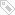标签：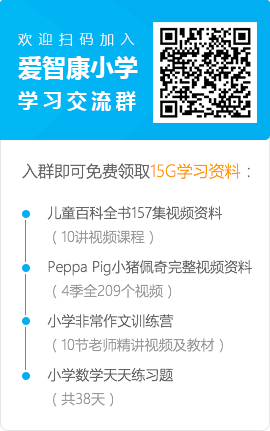• 全国课程在线咨询
• 咨询热线：4000-121-121
• 扫描注册有礼

• 让学习更有效